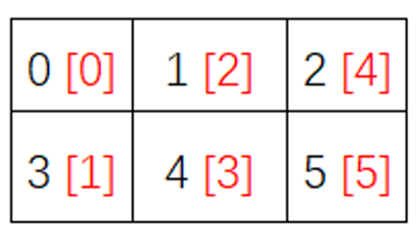## ndarray 对象的内部机理

### 1、ndarray 的组成

ndarray 与数组不同，它不仅仅包含数据信息，还包括其他描述信息。ndarray 内部由以下内容组成：

• 数据指针：一个指向实际数据的指针。
• 数据类型（dtype）：描述了每个元素所占字节数。
• 维度（shape）：一个表示数组形状的元组。
• 跨度（strides）：一个表示从当前维度前进道下一维度的当前位置所需要“跨过”的字节数。

NumPy 中，数据存储在一个均匀连续的内存块中，可以这么理解，NumPy 将多维数组在内部以一维数组的方式存储，我们只要知道了每个元素所占的字节数（dtype）以及每个维度中元素的个数（shape），就可以快速定位到任意维度的任意一个元素。

dtypeshape 前文中已经有详细描述，这里我们来讲下 strides

``ls = [[[1, 2, 3, 4], [5, 6, 7, 8], [9, 10, 11, 12]],       [[13, 14, 15, 16], [17, 18, 19, 20], [21, 22, 23, 24]]]a = np.array(ls, dtype=int)print(a)print(a.strides)``

``````[[[ 1  2  3  4]  [ 5  6  7  8]  [ 9 10 11 12]]

[[13 14 15 16]  [17 18 19 20]  [21 22 23 24]]](48, 16, 4)``````

## 普通迭代

ndarray 的普通迭代跟 Python 及其他语言中的迭代方式无异，N 维数组，就要用 N 层的 `for` 循环。

``````import numpy as np

ls = [[1, 2], [3, 4], [5, 6]]a = np.array(ls, dtype=int)for row in a:    for cell in row:        print(cell)``````

``123456``

## nditer 多维迭代器

NumPy 提供了一个高效的多维迭代器对象：nditer 用于迭代数组。在普通方式的迭代中，N 维数组，就要用 N 层的 `for` 循环。但是使用 `nditer` 迭代器，一个 `for` 循环就能遍历整个数组。（因为 ndarray 在内存中是连续的，连续内存不就相当于是一维数组吗？遍历一维数组当然只需要一个 `for` 循环就行了。）

### 1、基本示例

``ls = [[[1, 2, 3, 4], [5, 6, 7, 8], [9, 10, 11, 12]],      [[13, 14, 15, 16], [17, 18, 19, 20], [21, 22, 23, 24]]]a = np.array(ls, dtype=int)for x in np.nditer(a):    print(x, end=", ")``

``1, 2, 3, 4, 5, 6, 7, 8, 9, 10, 11, 12, 13, 14, 15, 16, 17, 18, 19, 20, 21, 22, 23, 24, ``

### 2、order 参数：指定访问元素的顺序

``ls = [[[1, 2, 3, 4], [5, 6, 7, 8], [9, 10, 11, 12]],      [[13, 14, 15, 16], [17, 18, 19, 20], [21, 22, 23, 24]]]a = np.array(ls, dtype=int, order='F')for x in np.nditer(a):    print(x, end=", ")``

``1, 13, 5, 17, 9, 21, 2, 14, 6, 18, 10, 22, 3, 15, 7, 19, 11, 23, 4, 16, 8, 20, 12, 24, ``

`nditer` 默认以内存中元素的顺序（order='K'）访问元素，对比例一可见，创建 ndarray 时，指定不同的顺序将影响元素在内存中的位置。

`nditer` 也可以指定使用某种顺序遍历。

``ls = [[[1, 2, 3, 4], [5, 6, 7, 8], [9, 10, 11, 12]],      [[13, 14, 15, 16], [17, 18, 19, 20], [21, 22, 23, 24]]]a = np.array(ls, dtype=int, order='F')for x in np.nditer(a, order='C'):    print(x, end=", ")``

``1, 2, 3, 4, 5, 6, 7, 8, 9, 10, 11, 12, 13, 14, 15, 16, 17, 18, 19, 20, 21, 22, 23, 24, ``

### 3、op_flags 参数：迭代时修改元素的值

``````import numpy as np

a = np.arange(5)for x in np.nditer(a, op_flags=['readwrite']):    x[...] = 2 * xprint(a)``````

``[0 1 2 3 4]``

### 4、flags 参数

`flags` 参数需要传入一个数组或元组，既然参数类型是数组，我原本以为可以传入多个值的，但是，就下面介绍的 4 种常用选项，我试了，不能传多个，例如 `flags=['f_index', 'external_loop']`，运行报错

#### （1）使用外部循环：external_loop

``````import numpy as np

ls = [[[1, 2, 3, 4], [5, 6, 7, 8], [9, 10, 11, 12]],      [[13, 14, 15, 16], [17, 18, 19, 20], [21, 22, 23, 24]]]a = np.array(ls, dtype=int, order='C')for x in np.nditer(a, flags=['external_loop'], order='C'):    print(x,)``````

``[ 1  2  3  4  5  6  7  8  9 10 11 12 13 14 15 16 17 18 19 20 21 22 23 24]``

``b = np.array(ls, dtype=int, order='F')for x in np.nditer(b, flags=['external_loop'], order='C'):    print(x,)``

``[1 2 3 4][5 6 7 8][ 9 10 11 12][13 14 15 16][17 18 19 20][21 22 23 24]``

#### （2）追踪索引：c_index、f_index、multi_index

``````import numpy as np

a = np.arange(6).reshape(2, 3)it = np.nditer(a, flags=['f_index'])

while not it.finished:    print("%d <%d>" % (it, it.index))    it.iternext()``````

``0 <0>1 <2>2 <4>3 <1>4 <3>5 <5>````````import numpy as np

a = np.arange(6).reshape(2, 3)it = np.nditer(a, flags=['multi_index'])

while not it.finished:    print("%d <%s>" % (it, it.multi_index))    it.iternext()``````

``0 <(0, 0)>1 <(0, 1)>2 <(0, 2)>3 <(1, 0)>4 <(1, 1)>5 <(1, 2)>``

### 5、同时迭代多个数组

``a = np.array([1, 2, 3], dtype=int, order='C')b = np.array([11, 12, 13], dtype=int, order='C')for x, y in np.nditer([a, b]):    print(x, y)``

``1 112 123 13``

## 其他函数

### 1、flatten函数

`flatten` 函数将多维 ndarray 展开成一维 ndarray 返回。

``flatten(order='C')``

``````import numpy as np

a = np.array([[1, 2, 3], [4, 5, 6]], dtype=int, order='C')b = a.flatten()print(b)print(type(b))``````

``[1 2 3 4 5 6]<class 'numpy.ndarray'>``

### 2、flat

`flat` 返回一个迭代器，可以遍历数组中的每一个元素。

``````import numpy as np

a = np.array([[1, 2, 3], [4, 5, 6]], dtype=int, order='C')for b in a.flat:    print(b)print(type(a.flat))``````

``123456<class 'numpy.flatiter'>``

## NumPy 超详细教程（3）：ndarray 的内部机理及高级迭代的更多相关文章

1. NumPy 超详细教程（2）：数据类型

系列文章地址 NumPy 最详细教程(1):NumPy 数组 NumPy 超详细教程(2):数据类型 NumPy 超详细教程(3):ndarray 的内部机理及高级迭代 文章目录 NumPy 数据类型 ...

2. NumPy 超详细教程（1）：NumPy 数组

系列文章地址 NumPy 最详细教程(1):NumPy 数组 NumPy 超详细教程(2):数据类型 NumPy 超详细教程(3):ndarray 的内部机理及高级迭代 文章目录 Numpy 数组:n ...

3. c++ 网络编程（九）LINUX/windows-IOCP模型 多线程超详细教程及多线程实现服务端

原文作者:aircraft 原文链接:https://www.cnblogs.com/DOMLX/p/9661012.html 先讲Linux下(windows下在后面可以直接跳到后面看): 一.线程 ...

4. Github上传代码菜鸟超详细教程【转】

最近需要将课设代码上传到Github上,之前只是用来fork别人的代码. 这篇文章写得是windows下的使用方法. 第一步:创建Github新账户 第二步:新建仓库 第三部:填写名称,简介(可选), ...

5. WebRTC VideoEngine超详细教程（三）——集成X264编码和ffmpeg解码

转自:http://blog.csdn.net/nonmarking/article/details/47958395 本系列目前共三篇文章,后续还会更新 WebRTC VideoEngine超详细教 ...

6. Struts2+Spring4+Hibernate4整合超详细教程

Struts2.Spring4.Hibernate4整合 超详细教程 Struts2.Spring4.Hibernate4整合实例-下载 项目目的: 整合使用最新版本的三大框架(即Struts2.Sp ...

7. 安装64位Oracle 10g超详细教程

安装64位Oracle 10g超详细教程 1. 安装准备阶段 1.1 安装Oracle环境 经过上一篇博文的过程,已经完成了对Linux系统的安装,本例使用X-Manager来实现与Linux系统的连 ...

8. 【python】10分钟教你用python打造贪吃蛇超详细教程

10分钟教你用python打造贪吃蛇超详细教程 在家闲着没妹子约, 刚好最近又学了一下python,听说pygame挺好玩的.今天就在家研究一下, 弄了个贪吃蛇出来.希望大家喜欢. 先看程序效果: 0 ...

9. 数学规划求解器lp_solve超详细教程

前言 最近小编学了运筹学中的单纯形法.于是,很快便按奈不住跳动的心.这不得不让我拿起纸和笔思考着,一个至关重要的问题:如何用单纯形法装一个完备的13? 恰巧,在我坐在图书馆陷入沉思的时候,一位漂亮的小 ...

## 随机推荐

1. 如何用plugman编辑和添加cordova插件

1.安装工具 进入nodejs, 安装工具plugman,管理插件,输入命令npm install -g plugman 等待下载安装 2.使用plugman命令生成插件框架 cmd 进入用于生成插件 ...

2. （三）SpringBoot基础篇- 持久层，jdbcTemplate和JpaRespository

一.介绍 SpringBoot框架为使用SQL数据库提供了广泛的支持,从使用JdbcTemplate的直接JDBC访问到完整的"对象关系映射"技术(如Hibernate).Spri ...

3. WinForm

参考文章:http://blog.csdn.net/clb929/article/list/7 用三层架构来做winform程序: http://blog.csdn.net/clb929/articl ...

4. C# DataGridView绑定List对象时，利用BindingList来实现增删查改

当DataGridView的DataSource是DataTable的时候,DataTable的数据改变时,DataGridView的数据会随之改变,无需重新绑定到DataGridView.  当Da ...

5. idea快捷

IntelliJ Idea 常用快捷键 列表(实战终极总结!!!!) 1. -----------自动代码-------- 常用的有fori/sout/psvm+Tab即可生成循环.System.ou ...

6. PHP生成腾讯云COS请求签名

目标 使用 PHP 创建 COS 接口所需要的请求签名 步骤 按照官方示例(也许是我笨,我怎么读都觉得官方文档结构费劲,示例细节互相不挨着,容易引起歧义),请求签名应用在需要身份校验的场景,即非公有读 ...

7. 2018 Unite大会——《使用UPA工具优化项目》演讲实录

2018年5月11日至13日,腾讯WeTest与Unity联合打造的移动游戏性能分析工具(Unity Performance Analysis,以下称为UPA)正式亮相2018 Unite大会,为Un ...

8. Can I use MyBatis to generate Dynamic SQL without executing it?

Although MyBatis was designed to execute the query after it builds it, you can make use of it's conf ...

9. JavaScript单线程和异步机制

随着对JavaScript学习的深入和实践经验的积累,一些原理和底层的东西也开始逐渐了解.早先也看过一些关于js单线程和事件循环的文章,不过当时看的似懂非懂,只留了一个大概的印象:浏览器中的js程序时 ...

10. 玩转Spring MVC(五)----在spring中整合log4j

在前边的基础上,本文主要总结一下如何在spring 中配置log4j,在本文末尾会给出完整项目的链接. 首先是web.xml中要新添加的代码: <!-- 6. 配置log4j --> &l ...